# 基于策略梯度的深度强化学习

## 基于价值函数学习的问题

### 重名情况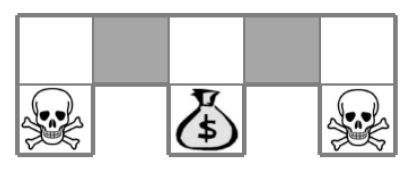### 解决

$$\pi_\theta(s,a)=\mathbb{P}[a|s,\theta]$$

## 策略目标函数

$$\begin{equation}J_1(\theta)=V_{\pi_\theta}(s_1)=\mathbb{E}_{\pi_\theta}[G_1]\label{policy_target_initial}\end{equation}$$

$$J_\textrm{avV}(\theta)=\sum_sd^{\pi_\theta}(s)V_{\pi_\theta}(s)$$

$$J_\textrm{avR}=\sum_sd^{\pi_\theta}(s)\sum_a{\pi_\theta}(s,a)R_s^a$$

$$\nabla_\theta{J(\theta)}=\begin{bmatrix} \frac{\partial J(\theta)}{\partial\theta_1}\\ \vdots\\ \frac{\partial J(\theta)}{\partial\theta_n} \end{bmatrix}$$

$$\Delta\theta=\alpha\nabla_\theta{J(\theta)}$$

$$J(\theta)=\mathbb{E}_{\pi_\theta}[r]=\sum_{s\in S}d(s)\sum_{a\in A}\pi_\theta(s,a)R_{s,a}$$

\begin{aligned}&\nabla_\theta J(\theta)=\sum_{s\in S}d(s)\sum_{a\in A}\nabla_\theta\pi_\theta(s,a)R_{s,a}\\ &=\sum_{s\in S}d(s)\sum_{a\in A}\pi_\theta(s,a)\nabla_\theta\log\pi_\theta(s,a)R_{s,a}\\ &=\mathbb{E}_{\pi_\theta}[\nabla_\theta\log\pi_\theta(s,a)r]\end{aligned}

$$\nabla_\theta J(\theta)=\mathbb{E}_{\pi_\theta}[\nabla_\theta\log\pi_\theta(s,a)Q_{\pi_\theta}(s,a)]$$

### 策略梯度的数学解释$^{}$

• $p(x;\theta)$：是参数化的概率分布函数。
• $f(x)$：在$p(x;\theta)$分布下的分值函数我们需要找到一个通过更新分布的参数$\theta$来提高采样的分值。

% <![CDATA[ \begin{align} \nabla_{\theta} E_x[f(x)] &= \nabla_{\theta} \sum_x p(x) f(x) & \textrm {definition of expectation} \\ & = \sum_x \nabla_{\theta} p(x) f(x) &\textrm{swap sum and gradient} \\ & = \sum_x p(x) \frac{\nabla_{\theta} p(x)}{p(x)} f(x) & \textrm{both multiply and divide by } p(x) \\ & = \sum_x p(x) \nabla_{\theta} \log p(x) f(x) & \textrm{use the fact that } \nabla_{\theta} \log(z) = \frac{1}{z} \nabla_{\theta} z \\ & = E_x[f(x) \nabla_{\theta} \log p(x) ] & \textrm{definition of expectation} \end{align}%]]

### 应用于离散行为空间的 Softmax 策略

$$\pi_\theta\propto e^{\phi(s,a)^T\theta}$$

$$\nabla_\theta\log\pi_\theta(s,a)=\phi(s,a)-\mathbb{E}_{\pi_\theta}[\phi(s,\cdot)]$$

### 应用于连续行为空间的高斯策略

$$\nabla\log\pi_\theta(s,a)=\frac{(a-\mu(s))\phi(s)}{\sigma^2}$$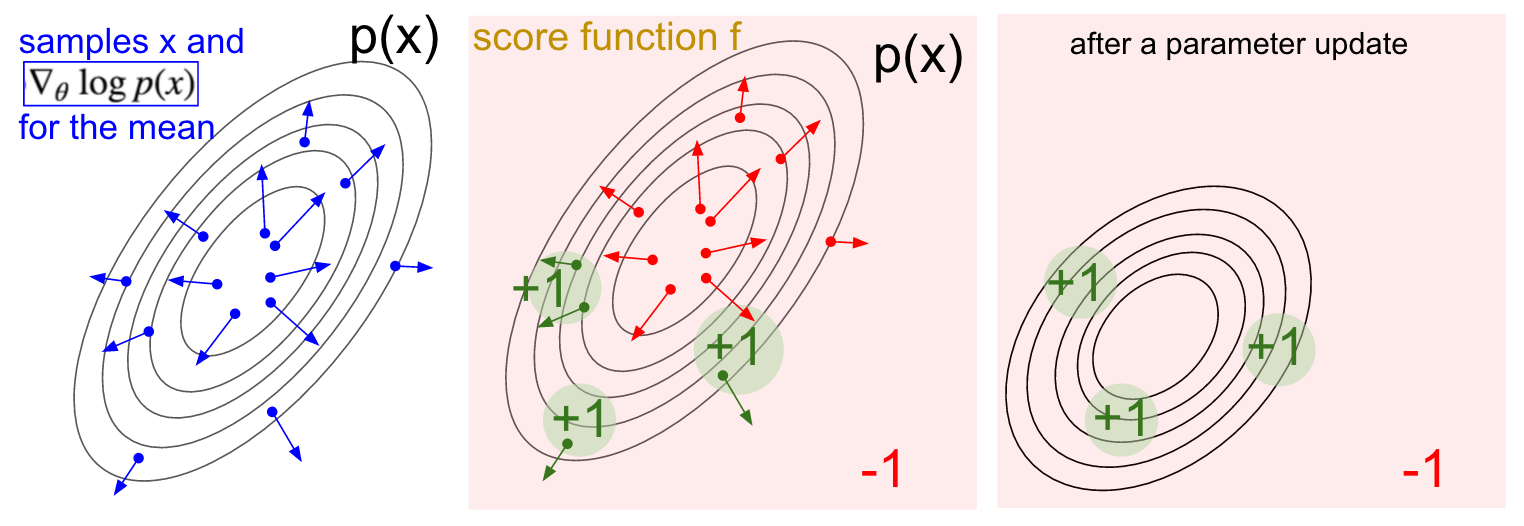## Actor-Critic 算法

Actor：可以理解为策略函数，生成行为与环境交互；Critic：行为价值函数，负责Actor的表现，并指导后续行为动作。Crtic的行为价值函数是基于策略$\pi_\theta$的一个近似：

$$Q_w(s,a)\approx Q_{\pi_\theta}(s,a)$$

$$\nabla_\theta J(\theta)\approx\mathbb{E}[\nabla_\theta\log\pi_\theta(s,a)Q_w(s,a)]\\ \Delta\theta=\alpha\nabla_\theta\log\pi_\theta(s,a)Q_w(s,a)$$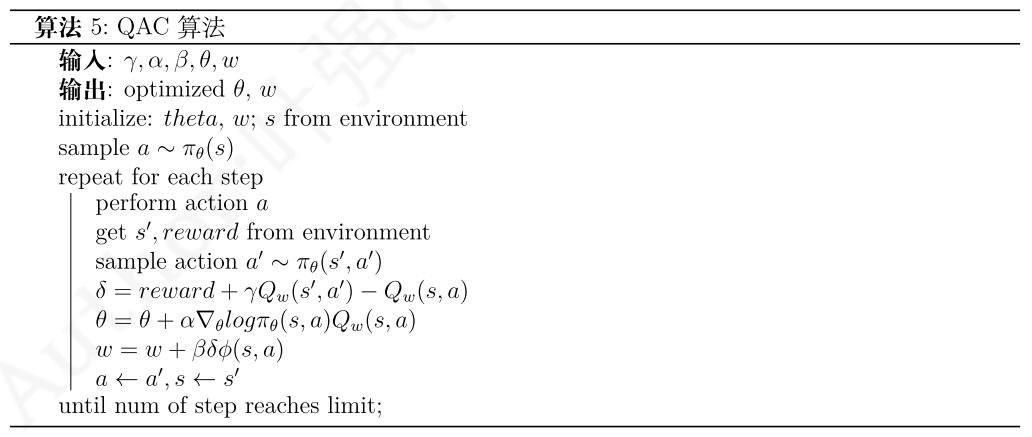1. 近似价值函数的梯度与分值函数的梯度相同，即$\nabla_wQ_w(s,a)=\nabla_\theta\log\pi_\theta(s,a)$
2. 近似价值函数的参数w能够最小化$\epsilon=\mathbb{E}_{\pi_\theta}[(Q_{\pi_\theta}-Q_w(s,a))^2]$

$$\nabla_\theta J(\theta)=\mathbb{E}_{\pi_\theta}[\nabla_\theta\log\pi_\theta(s,a)Q_w(s,a)]$$

$$\mathbb{E}_{\pi_\theta}[\nabla_\theta\log\pi_\theta(s,a)B(s)]=0$$

$$A_{\pi_\theta}(s,a)=Q_{\pi_\theta}(s,a)-V_{\pi_\theta}(s)$$

$$V_{\pi_\theta}(s)=\mathbb{E}_{\pi_\theta}[G_t|s_t=s]$$

$$\nabla_\theta J(\theta)=\mathbb{E}_{\pi_\theta}[\nabla_\theta\log\pi_\theta(s,a)A_{\pi_\theta}(s,a)]$$

\begin{aligned} & \mathbb{E}_{\pi_\theta}[\sigma_{\pi_\theta}|s,a]=\mathbb{E}_{\pi_\theta}[r+\gamma V_{\pi_\theta}(s^{'})|s,a]-V_{\pi_\theta}(s)\\ &= Q_{\pi_\theta}(s,a)-V_{\pi_\theta}(s)\\ &=A_{\pi_\theta}(s,a) \end{aligned}

$$\nabla_\theta J(\theta)=\mathbb{E}_{\pi_\theta}[\nabla_\theta\log\pi_\theta(s,a)\delta_{\pi_\theta}]$$

$$\delta_w=r+\gamma V_w(s^{'})-V_w(s)$$

MC $\Delta w=\alpha({\color{red}{G_t}}-V_w(s))\phi(s)$
TD(0)，红色部分为TD目标 $\Delta w=\alpha({\color{red}{r+\gamma V(s^{'})}}-V_w(s))\phi(s)$

1. MC：$\Delta\theta=\alpha(\color{red}{G_t}-V_w(s_t))\nabla_\theta\log\pi_\theta(s_t,a_t)$
2. TD(0)：$\Delta\theta=\alpha(\color{red}{r+\gamma V_w(s_{t+1})}-V_w(s_t))\nabla_\theta\log\pi_\theta(s_t,a_t)$
3. 前向$\text{TD}(\lambda)$：$\Delta\theta=\alpha(\color{red}{G_t^\lambda}-V_w(s_t))\nabla_\theta\log\pi_\theta(s_t,a_t)$
4. 后向$\text{TD}(\lambda)$，这个更新可以是在线(online)基于不完整的状态序列：$$\delta_t=r_{t+1}+\gamma V_w(s_{t+1})-V_w(s_t)\\e_t=\gamma\lambda e_{t-1}+\nabla\log\pi_\theta(s_t,a_t)\\\Delta\theta=\alpha\delta_t e_t$$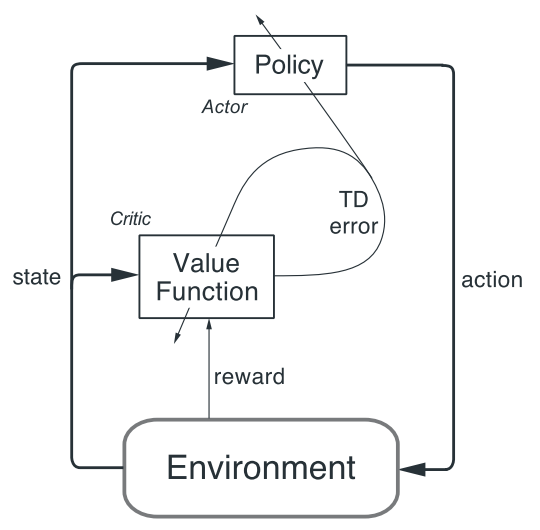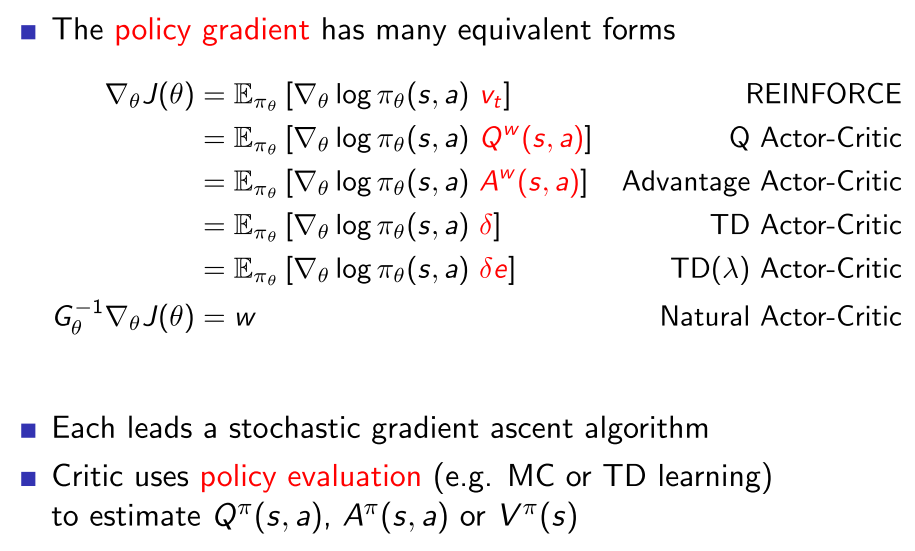## 异步强化学习算法$^{}$

### 并行(Parallel)的个体

$$\pi(a_t|s_t;\theta)$$

$$V(s_t|\theta_v)$$

$$\nabla_{\theta^{'}}\log\pi(a_t|s_t')A(s_t,a_t;\theta,\theta_v)$$

，其中优势函数$A(s_t,a_t;\theta,\theta_v)$的定义为：

$$\sum_{i=0}^{k-1}\gamma^it_{t+i}+\gamma^k V(s_{t+k};\theta_v)-V(s_t;\theta_v)$$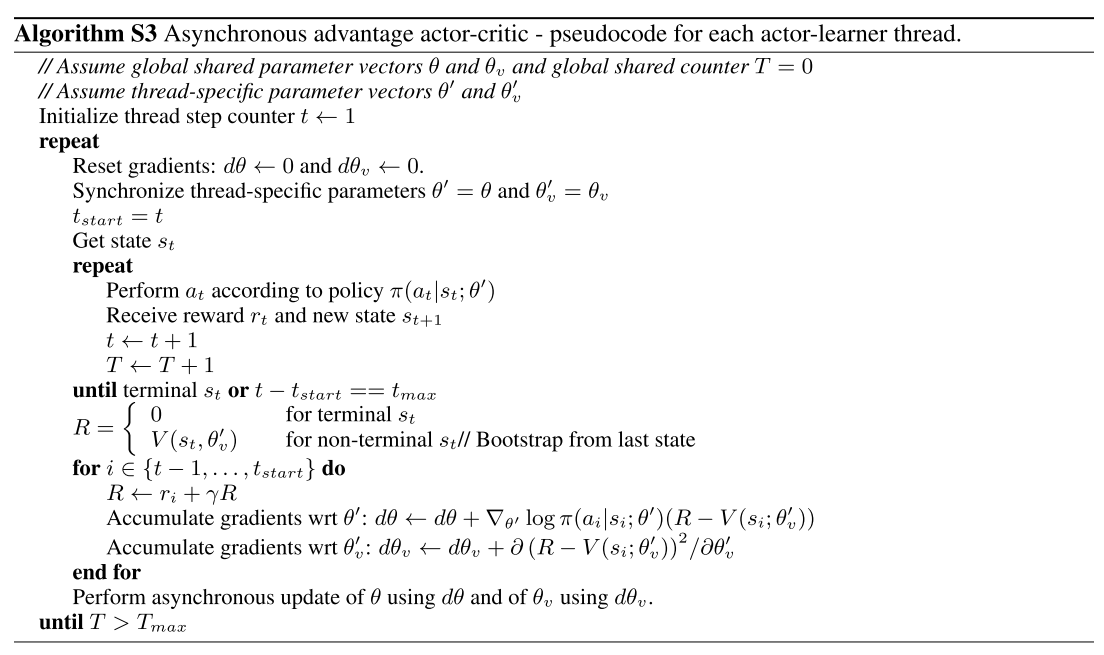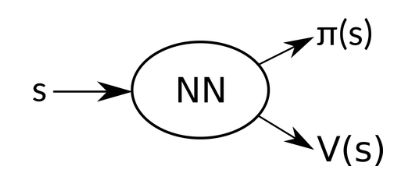$$\nabla_{\theta^{'}}\log\pi(a_t|s_t;\theta^{'})(G_t-V(s_t;\theta_v))+\beta\nabla_{\theta^{'}}H(\pi(s_t;\theta^{'}))$$

，其中H是信息熵。超参数(hyperparameter)$\beta$控制了h熵正则化的长度(entropy regularization term)。

### 实现的解释

A3C算法实现各个组件以及工作的流程：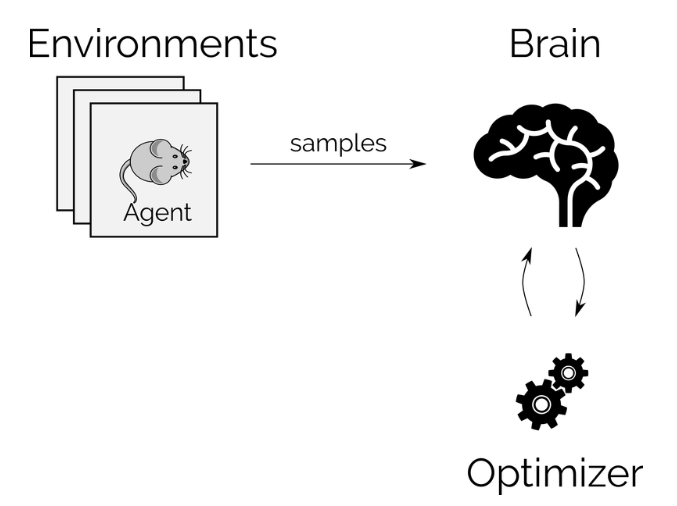# 补充：平稳分布

$$\pi=\pi \textbf{P}$$

Ergodic Markov chains有唯一的平稳分布Absorbing Markov Chains可能有多个元素非负的平稳分布。平稳分布刻画的是随机过程的稳定性(stability)，在有些情况下也能够表示马尔可夫的极限特征(?limiting behavior)

$$(\pi\textbf{P})^T=\pi^T\implies\textbf{P}^T\pi^T=\pi^T$$

$$\textbf{P}=\begin{bmatrix} 0.5 & 0.5 & 0\\ 0.25 & 0.5 & 0.5\\ 0 & 0.5 & 0.5 \end{bmatrix}$$

$$\det(\textbf{P}-\lambda\textbf{I})=\det\begin{bmatrix} 0.5-\lambda & 0.25 & 0\\ 0.5 & 0.5-\lambda & 0.5 \\ 0 & 0.25 & 0.5-\lambda \end{bmatrix}=0$$

0 (1,-2,1)
1 (1,2,1)
0.5 (-1,0,1)

# 参考&引用

1. Deep Reinforcement Learning: Pong from Pixels
2. (知乎)David Silver强化学习公开课中文讲解及实践-第七讲
3. (知乎)什么是无偏估计？
4. Stationary Distributions of Markov Chains
5. LET’S MAKE AN A3C: THEORY
6. Volodymyr Mnih, Adrià Puigdomènech Badia, Mehdi Mirza, Alex Graves, Timothy P. Lillicrap, Tim Harley, David Silver, Koray Kavukcuoglu. Asynchronous Methods for Deep Reinforcement Learning. arXiv:1602.01783, 2016.
7. (Github)avdmitry:rl_3d
8. Charles Beattie, Joel Z. Leibo, Denis Teplyashin, Tom Ward, Marcus Wainwright, Heinrich Küttler, Andrew Lefrancq, Simon Green, Víctor Valdés, Amir Sadik, Julian Schrittwieser, Keith Anderson, Sarah York, Max Cant, Adam Cain, Adrian Bolton, Stephen Gaffney, Helen King, Demis Hassabis, Shane Legg, Stig Petersen. DeepMind Lab. arXiv:1612.03801, 2016.
9. (Github)DeepMind Lab